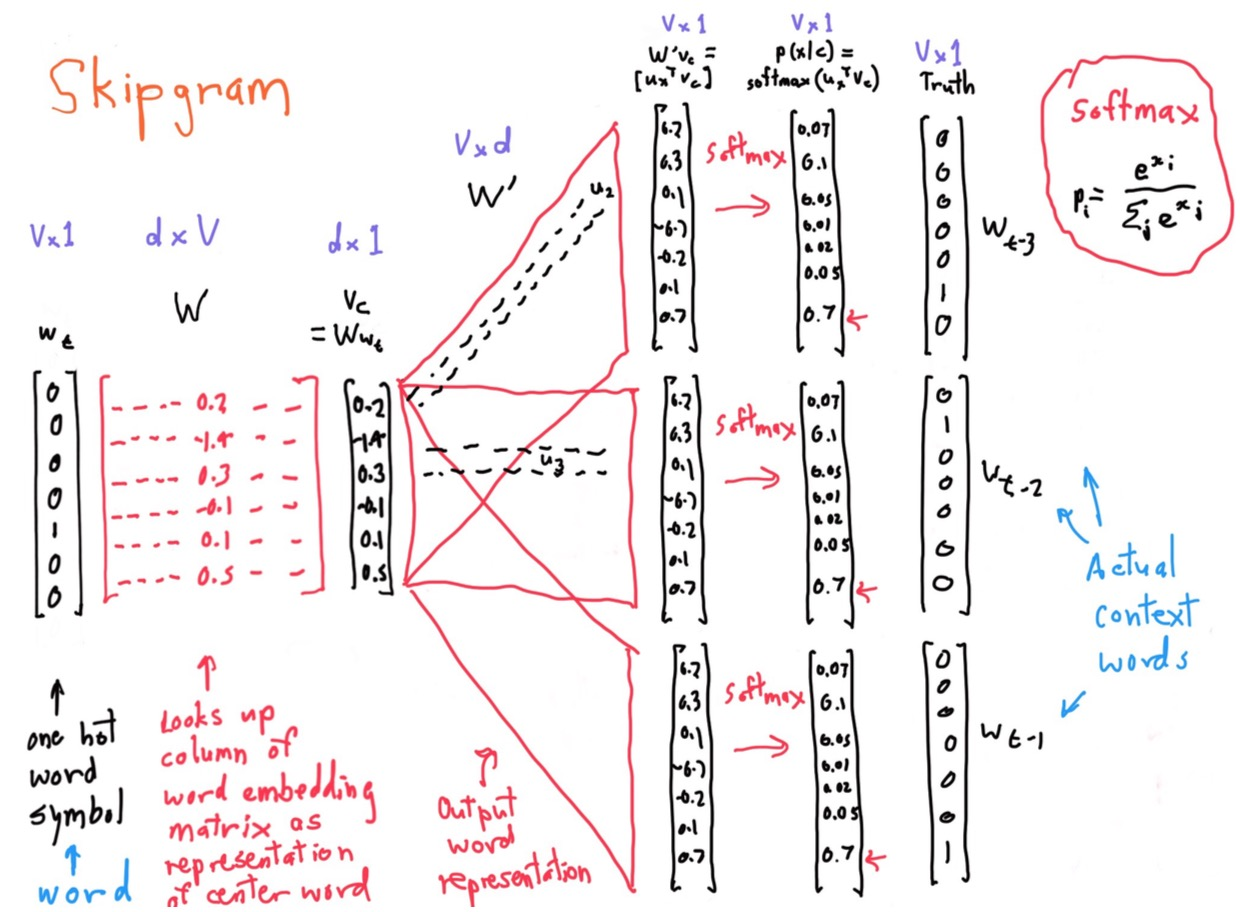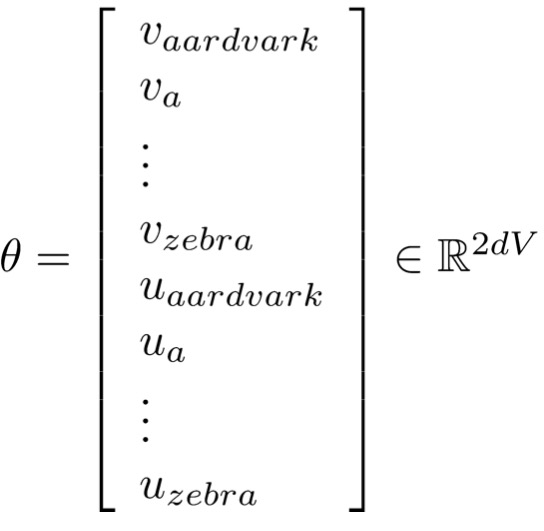Word Vectors 词向量

## 词义

### 检测

WordNet 是一个人工管理的词义库，可以用其来检测上下位 (is - a) 关系和近义词：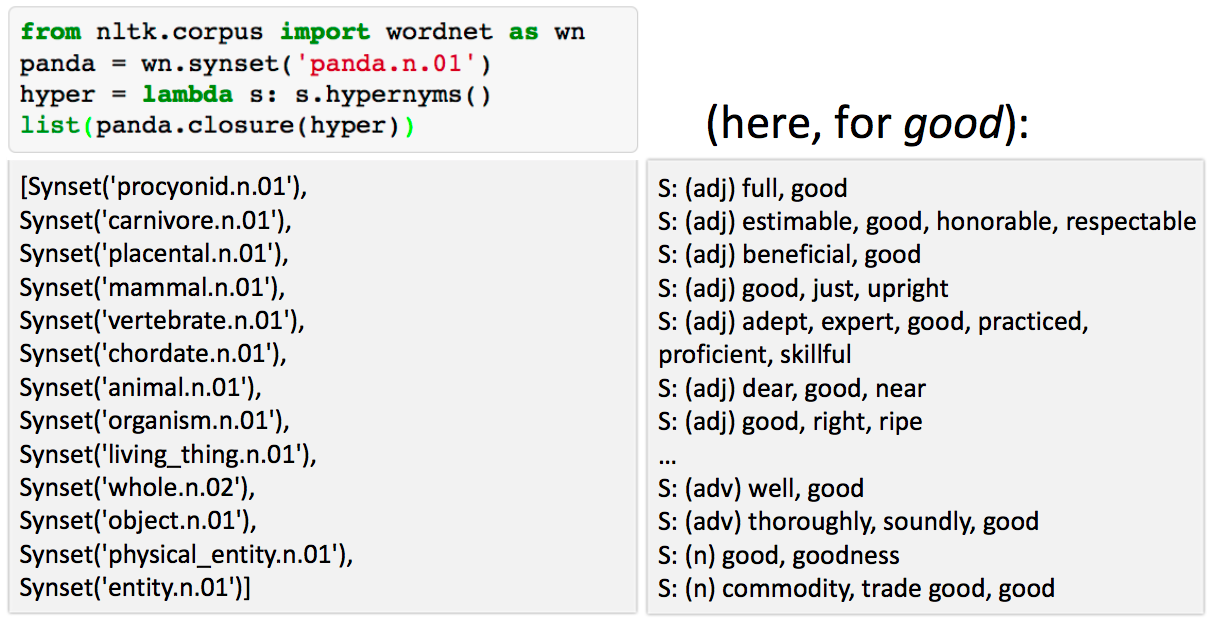• 需要人力进行维持，并无法一直保持更新；
• 属于主观的判断；
• 很难准确计算单次的相似性。

### 离散的表示方式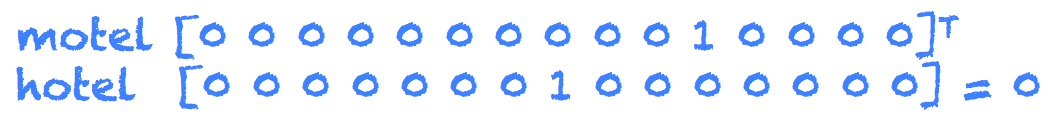### 基于离散相似性的表示方式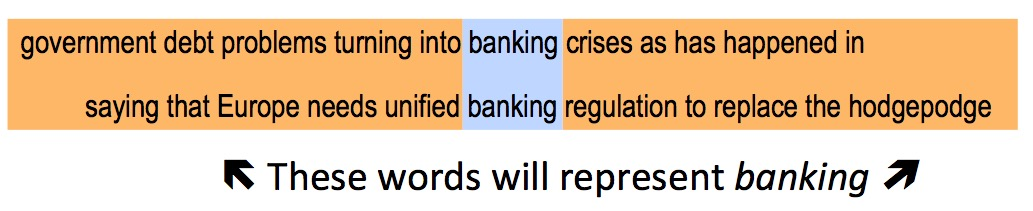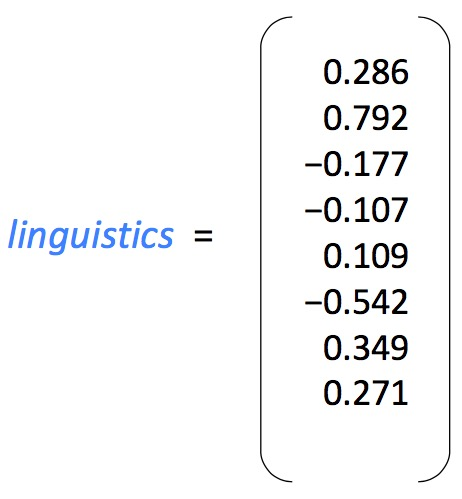### 词嵌入的学习神经网络

$$p(context|w_t)=…$$

$$J = 1 - p(w_{-t}|w_t)$$

## Word2vec

### 定义

Word2vec 可以预测每个单词的上下文词汇的概率。

### Skip-grams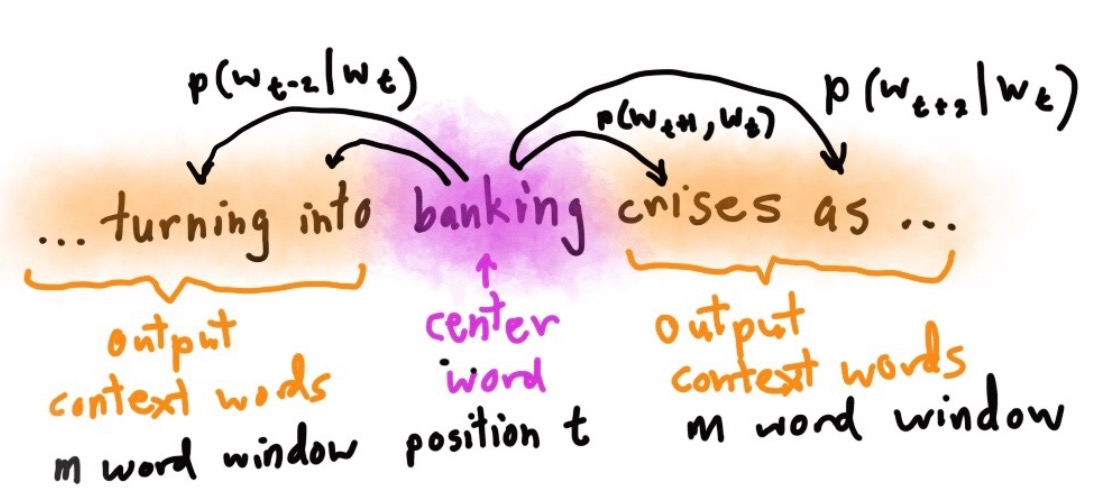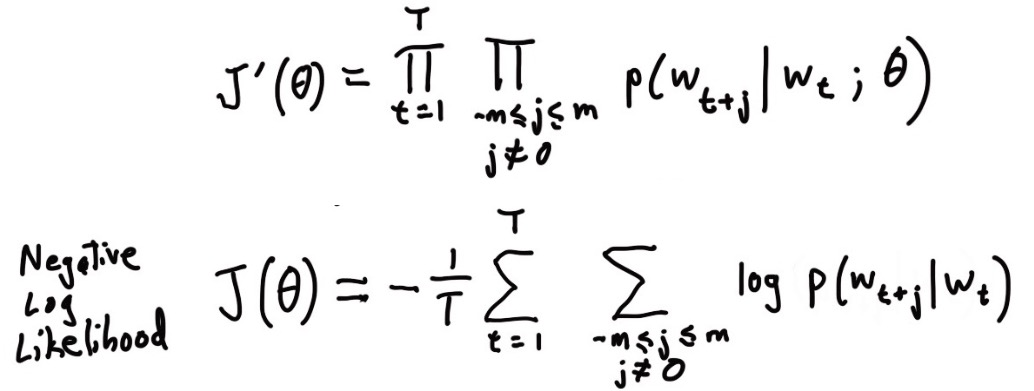#### Softmax 函数

Softmax 函数用于将 $R^v$ 空间映射成概率分布: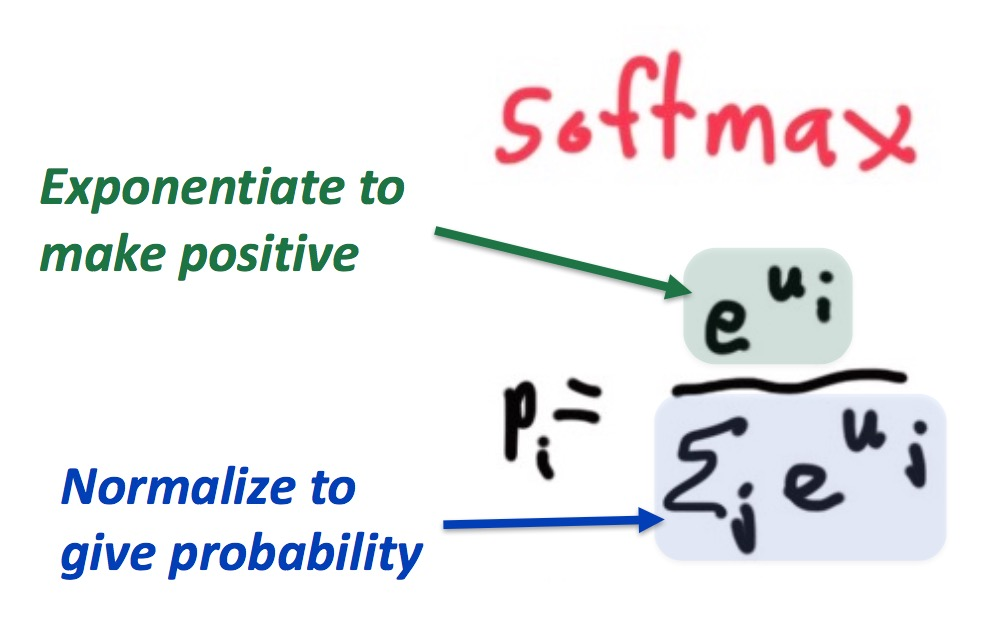#### 点积

$$u^Tv = u.v = \sum_{i=1}^nu_iv_i$$

#### Word2Vec

$$p(o|c) = \frac{exp(u_o^Tv_c)}{\sum_{w=1}^vexp(u_w^Tv_c)}$$